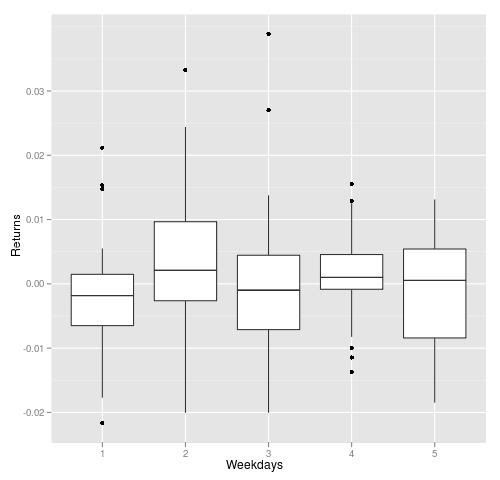# Returns on Easter week and one week after

March 21, 2010
By

Want to share your content on R-bloggers? click here if you have a blog, or here if you don't.

Inspired by CXO group report, I did a rerun of the same strategy on my data. Easter’s dates can be find at wikipedia. Overall, my results are similar to CXO group’s results.

In the graph below, I plotted daily returns on Easter week (Monday to Thursday) from 1982 to 2009. I prefer this way of showing things, where the range, minimum, maximum and mean of returns are presented.
It is clear, that only returns on Thursdays are above zero and t-test confirms that:
t = 2.235, df = 27, p-value = 0.03389. The rest is close to random.The graph below shows daily returns one week after Easter holidays. Although Monday looks like negative day, but it lacks significance:
t = -1.1517, df = 27, p-value = 0.2595 (should be less that 0.1).
The rest is noise.In summary, only returns on Thursdays have positive bias.

``` #R-Language code to repeat this test. require(quantmod) require(xts) easter<-as.matrix(read.table(file='data/easter.csv')) getSymbols('^GSPC',from='1980-01-01')   GSPC.delta<-Delt(log(Cl(GSPC))) GSPC.delta<-Delt(Cl(GSPC))   #returns during the week before Easter #returns on Thursdays nasa<-data.frame(as.double(GSPC.delta[(as.Date(easter,'%m/%d/%y')-3)]),4) colnames(nasa)<-c('ret','weekday')   #Monday to Wednesday for(i in 1:3) { tmp<-data.frame(as.double(GSPC.delta[(as.Date(easter,'%m/%d/%y')-(3+i))]),(4-i)) colnames(tmp)<-c('ret','weekday') rbind(nasa,tmp) nasa<-rbind(nasa,tmp) } t.test(nasa\$ret[nasa\$weekday==4])   require(ggplot2) qplot(factor(as.numeric(nasa\$week)),as.double(nasa\$ret),data=nasa,geom = "boxplot",ylab='Returns',xlab='Weekdays')   #returns during the week after Easter   GSPC.delta<-Delt(Cl(GSPC)) nasa<-data.frame(as.double(GSPC.delta[(as.Date(easter,'%m/%d/%y')+1)]),1) colnames(nasa)<-c('ret','weekday') for(i in 2:5) { print(i) tmp<-data.frame(as.double(GSPC.delta[(as.Date(easter,'%m/%d/%y')+i)]),i) colnames(tmp)<-c('ret','weekday') rbind(nasa,tmp) nasa<-rbind(nasa,tmp) } t.test(nasa\$ret[nasa\$weekday==1]);t.test(nasa\$ret[nasa\$weekday==2]) qplot(factor(as.numeric(nasa\$week)),as.double(nasa\$ret),data=nasa,geom = "boxplot",ylab='Returns',xlab='Weekdays') ```

R-bloggers.com offers daily e-mail updates about R news and tutorials about learning R and many other topics. Click here if you're looking to post or find an R/data-science job.
Want to share your content on R-bloggers? click here if you have a blog, or here if you don't.

Tags: , , ,